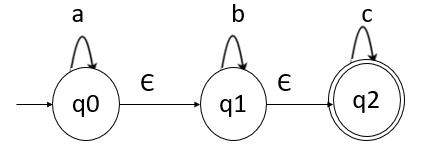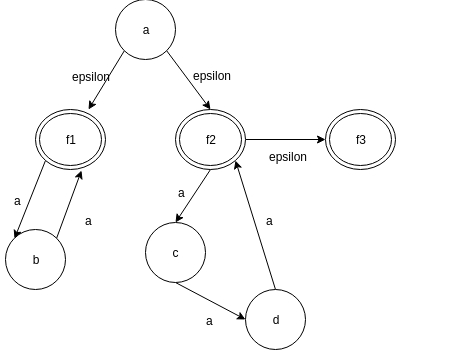# What is an epsilon closure in TOC?

Data Structure AlgorithmsComputer ScienceComputers

The ε closure(P) is a set of states which are reachable from state P on ε-transitions.

The epsilon closure is as mentioned below −

• ε-closure (P) = P, where P ∈ Q
• If there exists ε-closure (P) = {q} and 𝛿(q, ε) =r then, ε-closure (P) = {q, r}

## Example

Find ε-closure for the following Non-deterministic finite automata (NFA) with epsilon.## Solution

ε-closure (q0)= {q0,q1,q2}

self state+ ε-reachable states.

ε-closure (q1)= { q1,q2}

q1 is self-state and q2 is a state obtained from q1 with epsilon input.

ε-closure (q2)= {q2}

Lets us consider an example to understand more clear about epsilon closure −

Problem − find the number of epsilon closure in the below diagram?

Solution −The epsilon closure set of f2 consist of the elements − {f2, f3}. Therefore, the count of the element in the closure set is 2.